## Logical-Reasoning

 Question 1
A crime has been committed with four people at the scene of the crime. You are responsible for finding out who did it. You have recorded the following statements from the four witnesses, and you know one of them has committed the crime.
(1) Anuj says that Binky did it.
(2) Binky says that Anuj did it.
(3) Chacko says that Binky is telling the truth.
(4) Desmond says that Chacko is not lying.
You know that exactly three of the statements recorded are FALSE. Who committed the crime?
 A Anuj B Binky C Chacko D Desmond E Either Anuj or Binky; the information is insufficient to pinpoint the criminal
Logical-Reasoning       Logical-Reasoning       TIFR PHD CS & SS 2018
 Question 2
In the expression ‘Nothing is larger than itself’ the relation ‘is larger than’ is
 A antisymmetric B asymmetrical C intransitive D irreflexive
Logical-Reasoning       Relational-Argument       2012 June UGC NET Paper 1
 Question 3
Assertion (A) : There are more laws on the books today than ever before, and more crimes being committed than ever before.
Reason (R) : Because to reduce crime we must eliminate the laws.
Choose the correct answer from below :
 A (A) is true, (R) is doubtful and (R) is not the correct explanation of (A). B (A) is false, (R) is true and (R) is the correct explanation of (A). C (A) is doubtful, (R) is doubtful and (R) is not the correct explanation of (A). D (A) is doubtful, (R) is true and (R) is not the correct explanation of (A).
Logical-Reasoning       Assertion-and-Reason       2012 June UGC NET Paper 1
 Question 4
If the proposition “All men are not mortal” is true then which of the following inferences is correct ? Choose from the code given below :
1. “All men are mortal” is true.
2. “Some men are mortal” is false.
3. “No men are mortal” is doubtful.
4. “All men are mortal” is false.
 A 1, 2 and 3 B 2, 3 and 4 C 1, 3 and 4 D 1 and 3
Logical-Reasoning       Square-of-opposition       2012 June UGC NET Paper 1
Question 4 Explanation:Question 5
Determine the nature of the following definition :
“Abortion” means the ruthless murdering of innocent beings.
 A Lexical B Persuasive C Stipulative D Theoretical
Logical-Reasoning       Types-of-Definitions       2012 June UGC NET Paper 1
Question 5 Explanation: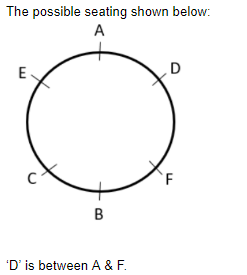Question 6
Which one of the following is not an argument ?
 A Devadutt does not eat in the day so he must be eating at night. B If Devadutt is growing fat and if he does not eat during the day, he will be eating at night. C Devadutt eats in the night so he does not eat during the day. D Since Devadutt does not eat in the day, he must be eating in the night.
Logical-Reasoning       Validity-of-Arguments       2012 June UGC NET Paper 1
Question 6 Explanation:
Argument: An exchange of diverging (or) opposite views.
→ Option B is not an argument.
 Question 7
Venn diagram is a kind of diagram to
 A represent and assess the validity of elementary inferences of syllogistic form. B represent but not assess the validity of elementary inferences of syllogistic form. C represent and assess the truth of elementary inferences of syllogistic form. D assess but not represent the truth of elementary inferences of syllogistic form
Logical-Reasoning       Venn-Diagram       2012 June UGC NET Paper 1
Question 7 Explanation:
Venn diagram is a kind of diagram to represent and assess the validity of elementary inferences of syllogistic form.
 Question 8
 A certainty B definite conclusion C predictive conjecture D surety
Logical-Reasoning       Analogical-Reasoning       2012 June UGC NET Paper 1
Question 8 Explanation:
Reasoning by analogy leads to predictive conjecture.
 Question 9
Which of the following statements are false ? Choose from the code given below :
1. Inductive arguments always proceed from the particular to the general.
2. A cogent argument must be inductively strong.
3. A valid argument may have a false premise and a false conclusion.
4. An argument may legitimately be spoken of as ‘true’ or ‘false’.
 A 2, 3 and 4 B 1 and 3 C 2 and 4 D 1 and 2
Logical-Reasoning       Types-of-Arguments       2012 June UGC NET Paper 1
 Question 10
A definition put forward to resolve a dispute by influencing attitudes or stirring emotions is called
 A Lexical B Persuasive C Stipulative D Precisions
Logical-Reasoning       Types-of-Definitions       2013 June UGC NET Paper 1
Question 10 Explanation:
Persuasive: Good at persuading someone to do (or) believe something through reasoning (or) the use of temptation.
 Question 11
Which of the codes given below contains only the correct statements?
Statements:
(i) Venn diagram is a clear method of notation.
(ii) Venn diagram is the most direct method of testing the validity of categorical syllogisms.
(iii) In Venn diagram method the premises and the conclusion of a categorical syllogism is diagrammed.
(iv) In Venn diagram method the three overlapping circles are drawn for testing a categorical syllogism.
 A (i), (ii) & (iii) B (i), (ii) & (iv) C (ii), (iii) & (iv) D (i), (iii) & (iv)
Logical-Reasoning       Venn-Diagram       2013 June UGC NET Paper 1
Question 11 Explanation:
Statements (i), (ii) & (iv) are true.
 Question 12
Inductive reasoning presupposes
 A Unity in human nature. B Integrity in human nature. C Uniformity in human nature. D Harmony in human nature.
Logical-Reasoning       Inductive-Reasoning       2013 June UGC NET Paper 1
Question 12 Explanation:
Inductive reasoning presupposes the uniformity in human nature.
 Question 13
If the statement ‘some men are honest’ is false, which among the following
statements will be true. Choose the correct code given below:
(i) All men are honest
(ii) No men are honest
(iii) Some men are not honest.
(iv) All men are dishonest.
 A (i), (ii) and (iii) B (ii), (iii) and (iv) C (i), (iii) and (iv) D (ii), (i) and (iv)
Logical-Reasoning       Square-of-opposition       2013 June UGC NET Paper 1
 Question 14
Given below is a diagram of three circles A, B and C over-lapping each other? The circle A represents the class of honest people, the circle B represent the class of sincere people and circle C represents the class of politicians. p, q, r, s, U, X, Y represent different regions. Select the code that represents the region indicating the class of honest politicians who are not sincere.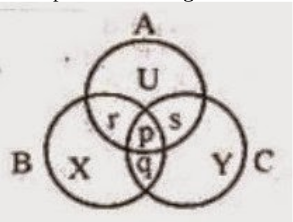A X B q C p D s
Logical-Reasoning       Venn-Diagram       2014 December UGC NET Paper 1
Question 14 Explanation:
‘S’ is the region of honest politicians who are not sincere.
 Question 15
“A man ought no more to value himself for being wiser than a woman if he owes his advantage to a better education, than he ought to boast of his courage for beating a man when his hands were tied.” The above passage is an instance of
 A Deductive argument B Hypothetical argument C Analogical argument D Factual argument
Logical-Reasoning       Analogical-Reasoning       2014 December UGC NET Paper 1
Question 15 Explanation:
The given passage is enhance of Analogical argument.
→ Analogical arguments (or argument by analogy) are a form of induction is derived from a comparison of similarities between the two are more cases.
 Question 16
By which of the following proposition, the proposition “wise men are hardly afraid of death” is contradicted?
 A Some wise men are afraid of death. B All wise men are afraid of death. C No wise men is afraid of death. D Some wise men are not afraid of death.
Logical-Reasoning       Square-of-opposition       2014 December UGC NET Paper 1
Question 16 Explanation:
Option ‘B’ is suitable contradicted to given proposition.
 Question 17
When in a group of propositions, one proposition is claimed to follow from the others, that group of propositions is called
 A An argument B A valid argument C An explanation D An invalid argument
Logical-Reasoning       Validity-of-Arguments       2014 December UGC NET Paper 1
Question 17 Explanation:
Argument:- Set of reasons given in support of an idea, theory (or) action.
 Question 18
Namita and Samita are brilliant and studious. Anita and karabi are obedient and irregular. Babita and Namita are irregular but brilliant. Samita and Kabita are regular and obedient. Who among them is/are brilliant, obedient, regular and studious?
 A Samita alone B Namita and Samita C Kabita alone D Anita alone
Logical-Reasoning       Venn-Diagram       2014 December UGC NET Paper 1
 Question 19
If two propositions having the same subject and predicate terms are such that one is the denial of the other, the relationship between them is called
 A Contradictory B Contrary C Sub-contrary D Sub-alteration
Logical-Reasoning       Square-of-opposition       2013 December UGC NET Paper 1
 Question 20
Ananaya and Krishna can speak and follow English. Bulbul can write and speak Hindi as Archana does. Archana talks with Ananya also in Bengali. Krishna can not follow Bengali. Bulbul talks with Ananya in Hindi. Who can speak and follow English, Hindi and Bengali?
 A Archana B Bulbul C Ananya D Krishna
Logical-Reasoning       Venn-Diagram       2013 December UGC NET Paper 1
 Question 21
A stipulative definition may be said to be
 A Always true B Always false C Sometimes true, sometimes false D Neither true nor false
Logical-Reasoning       Types-of-Definitions       2013 December UGC NET Paper 1
Question 21 Explanation:
Stipulative statement either said to be neither true (or) false.
 Question 22
When the conclusion of an argument follows from its premise/premises conclusively, the argument is called
 A Circular argument B Inductive argument C Deductive argument D Analogical argument
Logical-Reasoning       Deductive-Reasoning       2013 December UGC NET Paper 1
Question 22 Explanation:
Deductive arguments follows the premise/ premises conclusively and these premises are true then the conclusion must also be true.
 Question 23
Saturn and Mars are planets like the earth. They borrow light from the Sun and moves around the sun as the Earth does. So those planets are inhabited by various orders of creatures as the earth is
What type of argument is contained in the above passage?
 A Deductive B Astrological C Analogical D Mathematical
Logical-Reasoning       Analogical-Reasoning       2013 December UGC NET Paper 1
 Question 24
Given below are two premises. Four conclusions are drawn from those two premises in four codes. Select the code that states the conclusion validly drawn.
Premises:
(i) All saints are religious. (major)
(ii) Some honest persons are saints. (minor)
 A All saints are honest B Some saints are honest. C Some honest persons are religious. D All religious persons are honest
Logical-Reasoning       Validity-of-Arguments       2013 December UGC NET Paper 1
 Question 25
Yadav, Arjun, Rajesh and Kamal play cricket. Rajesh and Kamal play tennis but do not play football. Yadav does not play Badminton but play golf. Arjun does not play tennis. Kamal sometimes plays Badminton. Who does play only cricket ?
 A Yadav B Arjun C Rajesh D Kamal
Logical-Reasoning       Venn-Diagram       2013 September UGC NET Paper 1
 Question 26
A deductive argument can not be valid :
 A If its premise / premises is/are true and its conclusion is true. B If its premise / premises is /are true and its conclusion is false. C If its premise / premises is/are false and its conclusion is false. D If its premise / premises is / are false and its conclusion is true.
Logical-Reasoning       Deductive-Reasoning       2013 September UGC NET Paper 1
Question 26 Explanation:
Deductive argument is not valid when premises true and the conclusion is false.
Ans:- Option B
 Question 27
An analogical argument is strengthened by
 A making the claim bolder while its premises remain unchanged. B reducing the claim made on the basis of the premises affirmed. C remaining the claim unchanged while the evidence in its support is found to exhibit greater frailty. D None of the above.
Logical-Reasoning       Analogical-Reasoning       2013 September UGC NET Paper 1
Question 27 Explanation:
Option ‘B’ is appropriate to the analogical arguments.
 Question 28
If two propositions cannot both be false but may both be true, what is the relation between the two propositions ?
 A Contrary B Sub-contrary C Sub-alternation D Contradictory
Logical-Reasoning       Square-of-opposition       2013 September UGC NET Paper 1
Question 28 Explanation:
Subcontrary:- Two prepositions can be true, but cannot be false.
 Question 29
What is equivalent of the statement ‘All atheists are pessimists’ ?
 A All non-pessimists are nonatheists. B All non-atheists are nonpessimists. C All pessimists are atheists. D None of the above.
Logical-Reasoning       Square-of-opposition       2013 September UGC NET Paper 1
Question 29 Explanation:Question 30
"If a large diamond is cut up into little bits it will lose its value just as an army is divided up into small units of soldiers. It loses its strength." The argument put above may be called as
 A Analogical B Deductive C Statistical D Casual
Logical-Reasoning       Analogical-Reasoning       2014 June UGC NET Paper 1
Question 30 Explanation:
The given argument can be called as analogical.
→ Analogical arguments are a form of induction where a conclusion is derived from a comparison of similarities between two (or) more cases.
 Question 31
Given below are some characteristics of logical argument. Select the code which expresses a characteristic which is not of inductive in character.
 A The conclusion is claimed to follow from its premises. B The conclusion is based on causal relation. C The conclusion conclusively follows from its premises. D The conclusion is based on observation and experiment
Logical-Reasoning       Inductive-Reasoning       2014 June UGC NET Paper 1
Question 31 Explanation:
Inductive reasoning is a method of reasoning in which the premises are viewed as supplying some evidence for the truth of the conclusion and this is in contrast to deductive reasoning.
 Question 32
If two propositions having the same subject and predicate terms can both be true but cannot both be false, the relation between those two propositions is called
 A contradictory B contrary C subcontrary D subaltern
Logical-Reasoning       Square-of-opposition       2014 June UGC NET Paper 1
Question 32 Explanation:
Subcontrary:- Denoting the preposition can be true but cannot be false.
 Question 33
Given below are two premises and four conclusions drawn from those premises. Select the code that expresses conclusion drawn validly from the premises (separately or jointly).
Premises:
(a) All dogs are mammals.
(b) No cats are dogs.
Conclusions:
(i) No cats are mammals
(ii) Some cats are mammals.
(iii) No Dogs are cats
(iv) No dogs are non-mammals.
 A (i) only B (i) and (ii) C (iii) and (iv) D (ii) and (iii)
Logical-Reasoning       Validity-of-Arguments       2014 June UGC NET Paper 1
Question 33 Explanation:Question 34
Given below is a diagram of three circles A, B & C inter-related with each of Indians. The circle B represents the class of scientists and circle C represents the class of politicians. p,q,r,s... represent different regions. Select the code containing the region that indicates the class of Indian scientists who are not politicians.A q and s only B s only C s and r only D p, q and s only
Logical-Reasoning       Venn-Diagram       2014 June UGC NET Paper 1
 Question 35
If two propositions are connected in such a way that they cannot both be false although they may both be true, then their relationship is called
 A Contrary B Subcontrary C Contradictory D Subalternation
Logical-Reasoning       Square-of-opposition       2016 August NTA UGC NET Paper 1
Question 35 Explanation:
Sub contrary: Denoting prepositions which can both be true but cannot be false.
 Question 36
Which one among the following is not a characteristic of a deductive type of argument?
 A The conclusion follows from the premise/premises necessarily. B The argument admits degree of complexity. C The argument provides us knowledge about matters of fact. D The argument must be either valid or invalid.
Logical-Reasoning       Deductive-Reasoning       2016 August NTA UGC NET Paper 1
Question 36 Explanation:
A deductive argument is an argument that is intended by the arguer to be deductively valid that is to provide a guarantee of the truth of the conclusion provided that the argument's premises are true.
 Question 37
Select the code which states the condition of an invalid deductive argument:
 A All the premises are true but the conclusion is false. B Some of the premises are true but the conclusion is false. C All the premises are false and the conclusion is also false. D All the premises are true and the conclusion is also true.
Logical-Reasoning       Deductive-Reasoning       2016 August NTA UGC NET Paper 1
 Question 38
Given below are two premises, with four conclusions drawn from them (taking singly or together); which conclusions are validly drawn? Select the correct answer from the codes given below:
Premises:
(i) All bats are mammals.
(ii) Birds are not bats.
Conclusions:
(a) Birds are not mammals.
(b) Bats are not birds.
(c) All mammals are bats.
(d) Some mammals are bats.
 A (a), (b) and (d) B (b) and (d) C (a) and (c) D (b), (c) and (d)
Logical-Reasoning       Validity-of-Arguments       2016 August NTA UGC NET Paper 1
Question 38 Explanation:Question 39
When a definition implies a proposal to use the definiendum to mean what is meant by the definiens it is called
 A Lexical definition B Stipulative definition C Precising definition D Persuasive definition
Logical-Reasoning       Types-of-Definitions       2016 August NTA UGC NET Paper 1
 Question 40
Select the code which is not correct:
An analogical argument is strengthened by
 A Increasing the number of entities. B Increasing the number of similar respects. C Reducing the claim made earlier stronger. D Making the conclusion stronger when premises remain unchanged.
Logical-Reasoning       Analogical-Reasoning       2016 August NTA UGC NET Paper 1
 Question 41
Among the following statements two are contradictory to each other. Select the correct code that represents them:
Statements:
(a) All poets are philosophers.
(b) Some poets are philosophers.
(c) Some poets are not philosophers.
(d) No philosopher is a poet.
 A (b) and (c) B (a) and (b) C (a) and (d) D (a) and (c)
Logical-Reasoning       Square-of-opposition       2015 June NTA UGC Paper 1
Question 41 Explanation:
Statements (a) and (c) are contradictories which those statements gives the proper meaning for the contradictory.
 Question 42
When the purpose of a definition is to explain the use or to eliminate ambiguity the definition is called:
 A Persuasive B Stipulative C Theoretical D Lexical
Logical-Reasoning       Types-of-Definitions       2015 June NTA UGC Paper 1
Question 42 Explanation:
The word ‘Lexical’ is the most suitable for the given statement.
 Question 43
A deductive argument is invalid if:
 A Its premises and conclusions are all true B Its premises and conclusions are all false C Its premises are true but its conclusion is false D Its premises are false but its conclusion is true
Logical-Reasoning       Deductive-Reasoning       2015 June NTA UGC Paper 1
Question 43 Explanation:
In deductive arguments if premises are true then the conclusion is also true.
 Question 44
Which of the codes given below contains only the correct statements? Select the code:
Statements:
(a) Venn diagram represents the arguments graphically.
(b) Venn diagram can enhance our understanding.
(c) Venn diagram may be called valid or invalid.
(d) Venn diagram is clear method of notation.
 A (a), (c) and (d) B (a), (b) and (c) C (a), (b) and (d) D (b), (c) and (d)
Logical-Reasoning       Venn-Diagram       2015 June NTA UGC Paper 1
Question 44 Explanation:
Inductive reasoning gives knowledge of facts about the world.
 Question 45
Inductive reasoning is grounded on:
 A Harmony of nature B Integrity of nature C Unity of nature D Uniformity of nature
Logical-Reasoning       Inductive-Reasoning       2015 June NTA UGC Paper 1
Question 45 Explanation:
Inductive reasoning can be grounded on uniformity of nature which is most appropriate.
 Question 46
If we want to seek new knowledge of facts about the world, we must rely on reason of the type:
 A Physiological B Inductive C Deductive D Demonstrative
Logical-Reasoning       Inductive-Reasoning       2015 June NTA UGC Paper 1
Question 46 Explanation:
Venn diagram is always valid. So, Statement III is wrong.
 Question 47
Among the following propositions two are related in such a way that one is the denial of the other. Which are those propositions? Select the correct code:
Propositions:
(a) All women are equal to men
(b) Some women are equal to men
(c) Some women are not equal to men
(d) No women are equal to men
 A (a) and (b) B (a) and (d) C (c) and (d) D (a) and (c)
Logical-Reasoning       Square-of-opposition       2016 July NTA UGC NET Paper 1
 Question 48
Select the code, which is not correct in the context of deductive argument with two premises:
 A An argument with one true premise, one false premise and a false conclusion may be valid. B An argument with two true premises and a false conclusion may be valid. C An argument with one true premise, one false premise and a false conclusion may be valid. D An argument with two false premises and a false conclusion may be valid.
Logical-Reasoning       Deductive-Reasoning       2016 July NTA UGC NET Paper 1
 Question 49
Given below two premise and four conclusions are drawn from them (taking singly or together). Select the code that states the conclusion validly drawn.
Premises: (i) All religious persons are emotional.
(ii) Ram is a religious person.
Conclusion: (a) Ram is emotional.
(b) All emotional persons are religious.
(c) Ram is not a non-religious person.
(d) Some religious persons are not emotional.
 A (a), (b), (c) and (d) B (a) only C (a) and (c) only D (b) and (c) only
Logical-Reasoning       Validity-of-Arguments       2016 July NTA UGC NET Paper 1
Question 49 Explanation:Question 50
If the proposition ‘All thieves are poor’ is false, which of the following propositions can be claimed certainly to be true?
 A Some thieves are poor. B Some thieves are not poor. C No thief is poor D No poor person is a thief.
Logical-Reasoning       Square-of-opposition       2016 July NTA UGC NET Paper 1
Question 50 Explanation:
From the statement the suitable proposition is option ‘B’.
→ Some thieves are not poor which is correct.
 Question 51
Consider the following statement and select the correct code stating the nature of the argument involved in it:
To suppose that the earth is the only populated world in the infinite space is as absurd as to assert that in an entire field of millet only one grain will grow.
 A Astronomical B Anthropological C Deductive D Analogical
Logical-Reasoning       Analogical-Reasoning       2016 July NTA UGC NET Paper 1
Question 51 Explanation:
The given arguments are analogical.
 Question 52
Select the code, which is not correct about Venn diagram:
 A Venn diagram represents propositions as well as classes. B It can provide clear method of notation. C It can be either valid or invalid. D It can provide the direct method of testing the validity.
Logical-Reasoning       Venn-Diagram       2016 July NTA UGC NET Paper 1
Question 52 Explanation:
Venn diagrams are valid.
Option ‘C’ is not correct statement.
 Question 53
Consider the argument given below:
‘Pre - employment testing of teachers is quite fair because doctors, architects and engineers who are now employed had to face such a testing.’
What type of argument it is?
 A Deductive B Analogical C Psychological D Biological
Logical-Reasoning       Analogical-Reasoning       2015 December NTA UGC NET Paper 1
Question 53 Explanation:
The given arguments are analogical. Option ‘B’ is correct.
 Question 54
Among the following propositions two arc related in such a way that they can both be true although they cannot both be false. Which are those propositions? Select the correct code.
Propositions:
(a) Some priests are cunning.
(b) No priest is cunning.
(c) All priests are cunning.
(d) Some priests are not cunning.
 A (a) and (b) B (c) and (d) C (a) and (c) D (a) and (d)
Logical-Reasoning       Square-of-opposition       2015 December NTA UGC NET Paper 1
Question 54 Explanation:
Some priests are cunning and some are not cunning, these two statements are correct. Ans:- Option D
 Question 55
A Cluster of propositions with a structure that exhibits some inference is called
 A An inference B An argument C An explanation D A valid argument
Logical-Reasoning       Validity-of-Arguments       2015 December NTA UGC NET Paper 1
Question 55 Explanation:
Argument:- Set of reasons given in support of an idea, action (or) theory.
 Question 56
Consider the following assertion (A) and reason (R) and select the correct code given below:
(A): No man is perfect.
(R): Some men are not perfect.
 A Both (A) and (R) are true but (R) does not provide sufficient reason for (A). B Both (A) and (R) are true and (R) provides sufficient reason for (A). C (A) is true but (R) is false. D (A) is false but (R) is true.
Logical-Reasoning       Assertion-and-Reason       2015 December NTA UGC NET Paper 1
Question 56 Explanation:Question 57
A definition that has a meaning that is deliberately assigned to some symbol is called:
 A Lexical B Precising C Stipulative D Persuasive
Logical-Reasoning       Types-of-Definitions       2015 December NTA UGC NET Paper 1
 Question 58
If the proposition ‘No men are honest’ is taken to be false which of the following proposition/propositions can be claimed certainly to be true?
 A All men are honest B Some men are honest C Some men are not honest D No honest person is man
Logical-Reasoning       Square-of-opposition       2015 December NTA UGC NET Paper 1
 Question 59
Given below are some characteristics of reasoning. Select the code that states a characteristic which is not of deductive reasoning:
 A The conclusion must be based on observation and experiment. B The conclusion should be supported by the premise/premises. C The conclusion must follow from the premise/premises necessarily. D The argument may be valid or invalid.
Logical-Reasoning       Deductive-Reasoning       2017 January NTA UGC NET Paper 1
Question 59 Explanation:
Deductive reasoning is the presentation of statements that are assumed (or) known to be true as premises for a conclusion that necessarily followed by the statements.
 Question 60
Men and woman may have different reproductive strategies but neither can be considered inferior or superior to the other, any more than a bird's wings can be considered superior or inferior to a fish's fins. What type of argument it is ?
 A Biological B Physiological C Analogical D Hypothetical
Logical-Reasoning       Types-of-Arguments       2017 January NTA UGC NET Paper 1
Question 60 Explanation:
The given arguments are hypothetical. Answer:- Option D
 Question 61
Among the following, two statements are related in such a way that if one becomes undetermined, the other will also be undetermined. Select the code that refers to these two statements.
Statements:
(i) Every fruit is nutritious.
(ii) Fruits are hardly nutritious.
(iii) Fruits are mostly nutritious.
(iv) Some food items are nutritious.
 A (i) and (ii) B (ii) and (iii) C (iii) and (iv) D (i) and (iv)
Logical-Reasoning       Square-of-opposition       2018 July 22 NTA UGC NET Paper 1
Question 61 Explanation:
If statement (i) is undetermined the other will also be undetermined.
 Question 62
Given below are two premises (a) and (b). Four conclusions are drawn from them. Select the code that embodies validly drawn conclusion(s) (taking the premises individually or jointly).
Premises:
(a) All saints are noble.
(b) Some religious men are saints.
Conclusions:
(i) Some religious men are noble.
(ii) All religious men are noble.
(iii) Some saints are religious men.
(iv) All noble persons are saints.
 A (i) and (ii) B (ii) and (iii) C (i) and (iii) D (i) and (iv)
Logical-Reasoning       Validity-of-Arguments       2018 July 22 NTA UGC NET Paper 1
Question 62 Explanation:Question 63
Which one is not correct in the context of deductive and inductive reasoning?
 A A deductive argument makes the claim that its conclusion is supported by its premises conclusively. B A valid deductive argument may have all false premises and true conclusion. C An inductive argument claims the probability of its conclusion. D An inductive argument cannot provide us any new information about matters of fact.
Logical-Reasoning       Types-of-Reasoning       2018 July 22 NTA UGC NET Paper 1
Question 63 Explanation:
Inductive reasoning is a method of reasoning in which the premises are viewed as supplying some evidence for the truth of the conclusion. Option (d) is not correct.
 Question 64
When the purpose of the definition is to explain some established use of a term, the definition is called
 A Stipulative B Lexical C Persuasive D Theoretical
Logical-Reasoning       Types-of-Definitions       2018 July 22 NTA UGC NET Paper 1
 Question 65
Given below are four statements. Among them, two are related in such a way that they can both be true but they cannot both be false. Select the code that indicates those two statements:
Statements:
(a) Honest people never suffer.
(b) Almost all honest people do suffer.
(c) Honest people hardly suffer.
(d) Each and every honest person suffers.
 A (a) and (c) B (a) and (d) C (b) and (c) D (a) and (b)
Logical-Reasoning       Square-of-opposition       2017 November NTA UGC NET Paper 1
Question 65 Explanation:
Statements (b) and (c) are true and option ‘a’ have no relation with the other statements and option is ‘d’ is relating to each and every honest person. There is a combination between b & d and c & d.
 Question 66
It is Truism to say that no one was there when life first appeared on earth. Any assertion about life’s origin thus, should be treated as a theory.
 A A narrative B An argument C A conjecture D A historical explanation
Logical-Reasoning       Arguments       2017 November NTA UGC NET Paper 1
 Question 67
A deductive argument is invalid if:
 A Its premises and conclusion are all false B Its premises are all false but its conclusion is true C Its premises are all true but its conclusion is false D Its premises and conclusion are all true
Logical-Reasoning       Deductive-Reasoning       2017 November NTA UGC NET Paper 1
Question 67 Explanation:
A deductive argument is the presentation of statements that are assumed (or) known to be true as premises for a conclusion that necessarily follows from the statements.
 Question 68
Given below are two premises (a and b), from those two premises four conclusions (i), (ii), (iii) and (iv) are drawn. Select the code that states the conclusion/conclusions drawn validity (taking the premises singularly or jointly).
Premises:
(a) All bats are mammals.
(b) No birds are bats.
Conclusions:
(i) No birds are mammals.
(ii) Some birds are not mammals.
(iii) No bats are birds.
(iv) All mammals are bats.
 A (i) and (ii) only B (iii) only C (iii) and (iv) only D (i) only
Logical-Reasoning       Validity-of-Arguments       2017 November NTA UGC NET Paper 1
Question 68 Explanation:Question 69
Just melting ice-cubes do not causes a glass of water to overflow, melting sea – ice does not increase ocebic volume. What type of argument is it?
 A Hypothetical B Psychological C Statistical D Analogical
Logical-Reasoning       Analogical-Reasoning       2017 November NTA UGC NET Paper 1
 Question 70
Statements :
I All students are ambitious
II All ambitious persons are hard working
Conclusions :
(i) All students are hard-working
(ii) All hardly working people are not ambitious
Which of the following is correct ?
 A Only (i) is correct B Only (ii) is correct C Both (i) and (ii) are correct D Neither (i) nor (ii) is correct
Logical-Reasoning       Validity-of-Arguments       2005 Dec UGC NET Paper-1
Question 70 Explanation: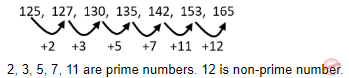Question 71
Statement :
Most students are intelligent
Conclusions :
(i) Some students are intelligent
(ii) All students are not intelligent
Which of the following is implied ?
 A Only (i) is implied B Only (ii) is implied C Both (i) and (ii) are implied D Neither (i) nor (ii) is implied
Logical-Reasoning       Validity-of-Arguments       2005 Dec UGC NET Paper-1
Question 71 Explanation:
Most students are intelligent means that not all students are intelligent. Only (ii) is implied.
 Question 72
Statement : Most labourers are poor
Conclusions :
(i) Some labourers are poor
(ii) All labourers are not poor
Which of the following is implied ?
 A Only (i) is implied B Only (ii) is implied C Both (i) and (ii) are implied D Neither (i) nor (ii) is implied
Logical-Reasoning       Validity-of-Arguments       2005 Dec UGC NET Paper-1
Question 72 Explanation:
Most labours are poor means that not all labours are poor. Only (ii) is implied.
 Question 73
The following question is based on the diagram given below. If the two small circles represent formal class-room education and distance education and the big circle stands for university system of education, which figure represents the university systems?
 A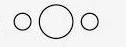B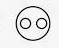C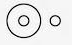D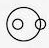Logical-Reasoning       Venn-Diagram       2008 June UGC NET Paper 1
 Question 74
The statement, 'To be non-violent is good' is a:
 A Moral judgement B Factual judgement C Religious judgement D Value judgement
Logical-Reasoning       Types-of-Judgement       2008 June UGC NET Paper 1
 Question 75
Assertion (A): Man is a rational being. Reason (R): Man is a social being.
 A Both (A) and (R) are true and (R) is the correct explanation of (A) B Both (A) and (R) are true but (R) is not the correct explanation of (A) C (A) is true but (R) is false D (A) is false but (R) is true
Logical-Reasoning       Assertion-and-Reason       2008 June UGC NET Paper 1
 Question 76
Value Judgements are:
 A Factual Judgements B Ordinary Judgements C Normative Judgements D Expression of public opinion
Logical-Reasoning       Types-of-Judgement       2008 June UGC NET Paper 1
 Question 77
Deductive reasoning proceeds from:
 A general to particular B particular to general C one general conclusion to another general conclusion D one particular conclusion to another particular conclusion
Logical-Reasoning       Deductive-Reasoning       2008 June UGC NET Paper 1
 Question 78
Deductive argument involves
 A sufficient evidence B critical thinking C seeing logical relations D repeated observation
Logical-Reasoning       Deductive-Reasoning       2009 December UGC NET Paper 1
Question 78 Explanation:
Deductive arguments follows the premises conclusively and these premises are true then the conclusion also be true which involves logical relations.
 Question 79
Inductive reasoning is based on or presupposes
 A uniformity of nature B God created the world C unity of nature D laws of nature
Logical-Reasoning       Inductive-Reasoning       2009 December UGC NET Paper 1
 Question 80
To be critical, thinking must be
 A practical B socially relevant C individually satisfying D analytical
Logical-Reasoning       Types-of-Definitions       2009 December UGC NET Paper 1
Question 80 Explanation:
To be critical, thinking must be socially relevant.
 Question 81
Which of the following is an analogous statement?
 A Man is like God B God is great C Gandhiji is the Father of the Nation D Man is a rational being.
Logical-Reasoning       Types-of-Arguments       2009 December UGC NET Paper 1
Question 81 Explanation:
Analogous:- Comparable in certain respects, typically in a way which makes clearer the nature of the things.
 Question 82
Questions from 35 - 36 are based on the following diagram in which there are three intersecting circles. H representing The Hindu, I representing Indian Express and T representing The Times of India. A total of 50 persons were surveyed and the number in the Venn diagram indicates the number of persons reading the newspapers.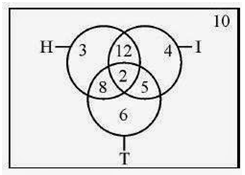How many persons would be reading at least two newspapers?
 A 23 B 25 C 27 D 29
Logical-Reasoning       Venn-Diagram       2009 December UGC NET Paper 1
Question 82 Explanation:
Persons reading atleast two papers is 8 + 12 + 5 + 2 = 27
 Question 83
Questions from 35 - 36 are based on the following diagram in which there are three intersecting circles. H representing The Hindu, I representing Indian Express and T representing The Times of India. A total of 50 persons were surveyed and the number in the Venn diagram indicates the number of persons reading the newspapers.How many persons would be reading almost two newspapers?
 A 23 B 25 C 27 D 48
Logical-Reasoning       Venn-Diagram       2009 December UGC NET Paper 1
Question 83 Explanation:
Almost two papers 8 + 12 + 5 - 2 = 23
 Question 84
Which of the following statements are mutually contradictory?
(i) All flowers are not fragrant.
(ii) Most flowers are not fragrant.
(iii) None of the flowers is fragrant.
(iv) Most flowers are fragrant.
Choose the correct answer from the code given below:
 A (i) and (ii) B (i) and (iii) C (ii) and (iii) D (iii) and (iv)
Logical-Reasoning       Square-of-opposition       2006 December UGC NET Paper 1
Question 84 Explanation:
(iii) and (iv) statements are mutually contradicted.
 Question 85
Which of the following statement/s are ALWAYS FALSE?
(i) The sun will not rise in the East some day.
(ii) A wooden table is not a table.
(iii) Delhi city will be drowned under water.
(iv) Cars run on water as fuel. Choose the correct answer from the code given below:
 A (i), (iii) and (iv) B Only (iii) C (i), (ii) and (iii) D (ii) alone
Logical-Reasoning       Logical-Reasoning       2006 December UGC NET Paper 1
Question 85 Explanation:
A wooden table can be a table which is not false all the cases. Ans:- Option A
 Question 86
Find out the number which should come at the place of question mark which will complete the following series: 5, 4, 9, 17, 35, ? = 139
 A 149 B 79 C 49 D 69
Logical-Reasoning       Venn-Diagram       2007 December UGC NET Paper 1
Question 86 Explanation:Question 87
Questions 22 to 24 are based on the following diagram in which there are three interlocking circles I, S and P, where circle I stands for Indians, circle S for Scientists and circle P for Politicians. Different regions in the figure are lettered from a to f• The region which represents Non-Indian Scientists who are Politicians:

 A f B d C a D c
Logical-Reasoning       Venn-Diagram       2007 December UGC NET Paper 1
Question 87 Explanation:
f → Non indian who are politicians and Indian scientists.
d → Indian scientists not politicians
a → Indian scientists and politicians
c → Only Indians neither politicians or neither scientist
Ans:- Option A
 Question 88
Questions 22 to 24 are based on the following diagram in which there are three interlocking circles I, S and P, where circle I stands for Indians, circle S for Scientists and circle P for Politicians. Different regions in the figure are lettered from a to f• The region which represents Indians who are neither Scientists nor Politicians:
 A g B c C f D a
Logical-Reasoning       Venn-Diagram       2007 December UGC NET Paper 1
Question 88 Explanation:
Refer Q.No:- 22
Ans: Option B
 Question 89
Questions 22 to 24 are based on the following diagram in which there are three interlocking circles I, S and P, where circle I stands for Indians, circle S for Scientists and circle P for Politicians. Different regions in the figure are lettered from a to f• The region which represents Politicians who are Indians as well as Scientists:
 A b B c C a D d
Logical-Reasoning       Venn-Diagram       2007 December UGC NET Paper 1
Question 89 Explanation:
Region ‘a’ represents all politicians, Indians and Scientists.
 Question 90
Logical arguments are based on:
 A Scientific reasoning B Customary reasoning C Mathematical reasoning D Syllogistic reasoning
Logical-Reasoning       Types-of-Arguments       2007 December UGC NET Paper 1
Question 90 Explanation:
Logical statements are based on syllogistic reasoning.
 Question 91
From the given four statements, select the two which cannot be true but yet both can be false.
Choose the right pair:
(i) All men are mortal
(ii) Some men are mortal
(iii) No man is mortal
(iv) Some men are not mortal
 A (i) and(ii) B (iii) and (iv) C (i) and(iii) D (ii) and(iv)
Logical-Reasoning       Square-of-opposition       2007 June UGC NET Paper 1
Question 91 Explanation:
If all men are not mortal statement II is false, if all men are mortal statement II can be false.
 Question 92
A Syllogism must have:
 A Three terms B Four terms C Six terms D Five terms
Logical-Reasoning       Deductive-inference-and-Syllogism       2007 June UGC NET Paper 1
Question 92 Explanation:
Syllogism:- A syllogism is a kind of logical argument that applies deductive reasoning to arrive at a conclusion based on two or more propositions that are asserted (or) assumed to be true. And syllogism must have four terms.
 Question 93
Copula is that part of proposition which denotes the relationship between:
 A Subject and predicate B Known and unknown C Major premise and minor premise D Subject and object
Logical-Reasoning       Proposition       2007 June UGC NET Paper 1
Question 93 Explanation:
Copula denotes relation between subject and predicate.
 Question 94
"E" denotes:
 A Universal Negative Proposition B Particular Affirmative Proposition C Universal Affirmative Proposition D Particular Negative Proposition
Logical-Reasoning       Proposition       2007 June UGC NET Paper 1
Question 94 Explanation:
“E” denotes universal negative proposition.
 Question 95
Circle graphs are used to show:
 A How various sections share in the whole? B How various parts are related to the whole? C How one whole is related to other wholes D How one part is related to other parts?
Logical-Reasoning       Venn-Diagram       2007 June UGC NET Paper 1
 Question 96
Logical reasoning is based on:
 A Truth of involved propositions B Valid relation among the involved propositions C Employment of symbolic language D Employment of ordinary language
Logical-Reasoning       Types-of-Reasoning       2008 December UGC NET Paper 1
 Question 97
Two propositions with the same subject and predicate terms but different in quality are:
 A Contradictory B Contrary C Subaltern D Identical
Logical-Reasoning       Square-of-opposition       2008 December UGC NET Paper 1
 Question 98
The premises of a valid deductive argument:
 A Provide some evidence for its conclusion B Provide no evidence for its conclusion C Are irrelevant for its conclusion D Provide conclusive evidence for its conclusion
Logical-Reasoning       Deductive-Reasoning       2008 December UGC NET Paper 1
 Question 99
Syllogistic reasoning is:
 A Deductive B Inductive C Experimental D Hypothetical
Logical-Reasoning       Deductive-Inference-and-Syllogism       2008 December UGC NET Paper 1
 Question 100
Study the following Venn diagram and answer questions nos. 31 to 33. Three circles representing GRADUATES, CLERKS and GOVERNMENT EMPLOYEES are intersecting. The intersections are marked A, B, C, e, f, g and h. Which part best represents the statements in questions 31 to 33?• Some Graduates are Government employees but not as Clerks.
 A h B g C f D e
Logical-Reasoning       Venn-Diagram       2008 December UGC NET Paper 1
 Question 101
Study the following Venn diagram and answer questions nos. 31 to 33. Three circles representing GRADUATES, CLERKS and GOVERNMENT EMPLOYEES are intersecting. The intersections are marked A, B, C, e, f, g and h. Which part best represents the statements in questions 31 to 33?• Clerks who are graduates as well as government employees:
 A e B f C g D h
Logical-Reasoning       Venn-Diagram       2008 December UGC NET Paper 1
 Question 102
Study the following Venn diagram and answer questions nos. 31 to 33. Three circles representing GRADUATES, CLERKS and GOVERNMENT EMPLOYEES are intersecting. The intersections are marked A, B, C, e, f, g and h. Which part best represents the statements in questions 31 to 33?• Some graduates are Clerks but not Government employees.
 A f B g C h D e
Logical-Reasoning       Venn-Diagram       2008 December UGC NET Paper 1
 Question 103
In a deductive argument conclusion is:
 A Summing up of the premises B Not necessarily based on premises C Entailed by the premises D Additional to the premises
Logical-Reasoning       Deductive-Reasoning       2009 June UGC NET Paper 1
Question 103 Explanation:
Deductive argument conclusion is entailed by the premises.
 Question 104
'No man are mortal' is contradictory of:
 A Some man are mortal B Some man are not mortal C All men are mortal D No mortal is man
Logical-Reasoning       Square-of-opposition       2009 June UGC NET Paper 1
Question 104 Explanation:Question 105
A deductive argument is valid if:
 A premises are false and conclusion true B premises are false and conclusion is also false C premises are true and conclusion is false D premises are true and conclusion is true
Logical-Reasoning       Deductive-Reasoning       2009 June UGC NET Paper 1
Question 105 Explanation:
Deductive argument valid if premises true and the conclusion is also true which is invalid when premises true and the conclusion false. Option (D) is correct.
 Question 106
Structure of logical argument is based on:
 A Formal validity B Material truth C Linguistic expression D Aptness of examples
Logical-Reasoning       Types-of-Arguments       2009 June UGC NET Paper 1
Question 106 Explanation:
Structure of logical argument is based on formal validity.
 Question 107
Questions 31 and 32 are based on the following venn diagram in which there are three intersecting circles representing Hindi knowing persons, English knowing persons and persons who are working as teachers. Different regions so obtained in the figure are marked as a, b, c, d, e, f and g.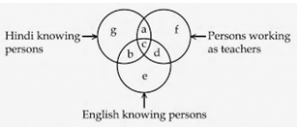• If you want to select Hindi and English knowing teachers, which of the following is to be selected?

 A g B b C c D e
Logical-Reasoning       Venn-Diagram       2009 June UGC NET Paper 1
Question 107 Explanation:
Region ‘c’ is most suitable as compared to region ‘b’.
 Question 108
Questions 31 and 32 are based on the following venn diagram in which there are three intersecting circles representing Hindi knowing persons, English knowing persons and persons who are working as teachers. Different regions so obtained in the figure are marked as a, b, c, d, e, f and g.• If you want to select persons, who do not know English and are not teachers, which of the region is to be selected?
 A e B g C b D a
Logical-Reasoning       Venn-Diagram       2009 June UGC NET Paper 1
Question 108 Explanation:
‘g’ is the region who don’t know english and not teaches.
 Question 109
In an examination, 35% of the total students failed in Hindi, 45% failed in English and 20% in both. The percentage of those who passed in both subjects is
 A 10 B 20 C 30 D 40
Logical-Reasoning       Venn-Diagram       2010 December UGC NET Paper 1
 Question 110
Two statements I and II given below are followed by two conclusions (a) and (b). Supposing the statements are true, which of the following conclusions can logically follow ? Statements:
Some flowers are red.
Some flowers are blue.
Conclusions:
(a) Some flowers are neither red nor blue.
(b) Some flowers are both red and blue.
 A Only (a) follows. B Only (b) follows. C Both (a) and (b) follow. D Neither (a) nor (b) follows.
Logical-Reasoning       Validity-of-Arguments       2010 December UGC NET Paper 1
Question 110 Explanation:
Some flowers may red and some may blue but both are not both red and blue. So option (b) fails.
→ Option (a) also fails we don't guarantee that some flowers neither red nor blue.
 Question 111
If the statement ‘all students are intelligent’ is true, which of the following statements are false?
(i) No students are intelligent.
(ii) Some students are intelligent.
(iii) Some students are not intelligent.
 A (i) and (ii) B (i) and (iii) C (ii) and (iii) D (i) only
Logical-Reasoning       Square-of-opposition       2010 December UGC NET Paper 1
Question 111 Explanation: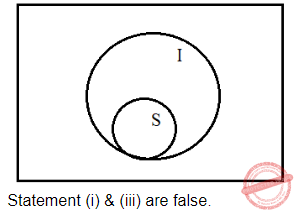Question 112
A reasoning where we start with certain particular statements and conclude with a universal statement is called
 A Deductive Reasoning B Inductive Reasoning C Abnormal Reasoning D Transcendental Reasoning
Logical-Reasoning       Inductive-Reasoning       2010 December UGC NET Paper 1
Question 112 Explanation:
Inductive reasoning is a method of reasoning in which the premises are viewed as supplying evidence for a conclusion which is also be universal.
 Question 113
If two small circles represent the class of the ‘men’ and the class of the ‘plants’ and the big circle represents ‘mortality’, which one of the following figures represent the proposition ‘All men are mortal.’ ?
 A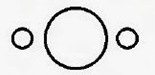B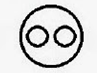C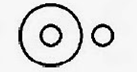DLogical-Reasoning       Venn-Diagram       2010 December UGC NET Paper 1
Question 113 Explanation: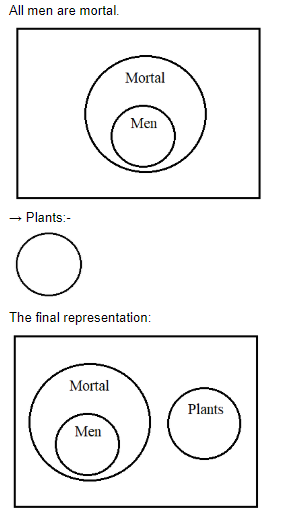Question 114
Two statements I and II given below are followed by two conclusions (a) and (b). Supposing the statements are true, which of the following conclusions can logically follow?
Some religious people are morally good.
Some religious people are rational.
Conclusions:
(a) Rationally religious people are good morally.
(b) Non-rational religious persons are not morally good.
 A Only (a) follows. B Only (b) follows. C Both (a) and (b) follow. D Neither (a) nor (b) follows.
Logical-Reasoning       Validity-of-Arguments       2010 June UGC NET Paper 1
 Question 115
Certainty is
 A an objective fact B emotionally satisfying C logical D ontological
Logical-Reasoning       Types-of-Definitions       2010 June UGC NET Paper 1
 Question 116
Questions  from  35 to 36 are  based on  the  following  diagram in  which there are  three  intersecting  circles I, S  and  P  where circle  I  stands  for Indians, circle S stands for scientists and circle P for politicians. Different regions of the figure are lettered from a to g.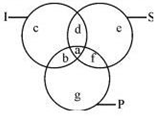• The region which represents non Indian scientists who are politicians.
 A f B d C a D c
Logical-Reasoning       Venn-Diagram       2010 June UGC NET Paper 1
 Question 117
Questions  from  35 to 36 are  based on  the  following  diagram in  which there are  three  intersecting  circles I, S  and  P  where circle  I  stands  for Indians, circle S stands for scientists and circle P for politicians. Different regions of the figure are lettered from a to g.• The region which represents politicians who are Indians as well as scientists.
 A b B c C a D d
Logical-Reasoning       Venn-Diagram       2010 June UGC NET Paper 1
 Question 118
Assertion (A): The coin when flipped next time will come up tails.
Reason (R): Because the coin was flipped five times in a row, and each time it came up heads.
Choose the correct answer from below:
 A Both (A) and (R) are true, and (R) is the correct explanation of (A). B Both (A) and (R) are false, and (R) is the correct explanation of (A). C (A) is doubtful, (R) is true, and (R) is not the correct explanation of (A). D (A) is doubtful, (R) is false, and (R) is the correct explanation of (A).
Logical-Reasoning       Assertion-and-Reason       2011 December UGC NET Paper 1
Question 118 Explanation:
(A) is doubtful, no guarantee that next time will come up tails.
(R) is false, sixth time there is a chance of getting both head and tail and (R) is not the correct explanation of (A).
 Question 119
The relation ‘is a sister of ’ is
 A non-symmetrical B symmetrical C asymmetrical D transitive
Logical-Reasoning       Relational-Argument       2011 December UGC NET Paper 1
 Question 120
If the proposition “Vegetarians are not meat eaters” is false, then which of the following inferences is correct? Choose from the codes given below:
1. “Some vegetarians are meat eaters” is true.
2. “All vegetarians are meat eaters” is doubtful.
3. “Some vegetarians are not meat eaters” is true.
4. “Some vegetarians are not meat eaters” is doubtful.
Codes:
 A 1, 2 and 3 B 2, 3 and 4 C 1, 3 and 4 D 1, 2 and 4
Logical-Reasoning       Square-of-opposition       2011 December UGC NET Paper 1
Question 120 Explanation:
Vegetarians are not meat eaters means that vegetarians are meat eaters.
Statement 1, 2, 3 are correct. There is no guarantee that some vegetarians are not meant eaters.
 Question 121
Determine the nature of the following definition: ‘Poor’ means having an annual income of Rs. 10,000.
 A persuasive B precising C lexical D stipulative
Logical-Reasoning       Types-of-Definitions       2011 December UGC NET Paper 1
Question 121 Explanation:
Precise means summarize. And this is most suitable for giving statement.
 Question 122
Which one of the following is not an argument?
 A If today is Tuesday, tomorrow will be Wednesday. B Since today is Tuesday, tomorrow will be Wednesday. C Ram insulted me so I punched him in the nose. D Ram is not at home, so he must have gone to town.
Logical-Reasoning       Validity-of-Arguments       2011 December UGC NET Paper 1
Question 122 Explanation:
Option A is not an argument.
 Question 123
Venn diagram is a kind of diagram to
 A represent and assess the truth of elementary inferences with the help of Boolean Algebra of classes. B represent and assess the validity of elementary inferences with the help of Boolean Algebra of classes. C represent but not assess the validity of elementary inferences with the help of Boolean Algebra of classes. D assess but not represent the validity of elementary inferences with the help of Boolean Algebra of classes.
Logical-Reasoning       Venn-Diagram       2011 December UGC NET Paper 1
Question 123 Explanation:
Venn diagram is a kind of diagram to represent and assess the validity of elementary inferences with the help of boolean algebra of classes.
 Question 124
Inductive logic studies the way in which a premise may
 A support and entail a conclusion B not support but entail a conclusion C neither support nor entail a conclusion D support a conclusion without entailing it
Logical-Reasoning       Inductive-Reasoning       2011 December UGC NET Paper 1
Question 124 Explanation:
Inductive reasoning is a method of reasoning in which the premises are viewed as supplying evidence for a conclusion.
→ Inductive logic supports a conclusion without entailing it.
 Question 125
Which of the following statements are true? Choose from the codes given below.
1. Some arguments, while not completely valid, are almost valid.
2. A sound argument may be invalid.
3. A cogent argument may have a probably false conclusion.
4. A statement may be true or false.
Codes:
 A 1 and 2 B 1, 3 and 4 C 4 alone D 3 and 4
Logical-Reasoning       Types-of-Arguments       2011 December UGC NET Paper 1
Question 125 Explanation:
Statement 3 and 4 are true.
 Question 126
If the statement ‘some men are cruel’ is false, which of the following statements/statement are/is true ?
(i) All men are cruel.
(ii) No men are cruel.
(iii) Some men are not cruel.
 A (i) and (iii) B (i) and (ii) C (ii) and (iii) D (iii) only
Logical-Reasoning       Square-of-opposition       2011 June UGC NET Paper 1
Question 126 Explanation:
Some men are cruel is false which means that some men are not cruel.
 Question 127
Consider the Assertion–I and Assertion–II and select the right code given below:
Assertion–I: Even Bank-lockers are not safe. Thieves can break them and take away your wealth. But thieves cannot go to heaven. So you should keep your wealth in heaven.
Assertion–II: The difference of skin-colour of beings is because of the distance from the sun and not because of some permanent traits. Skin-colour is the result of body’s reaction to the sun and its rays.
 A Both the assertions-I and II are forms of argument. B The assertion-I is an argument but the assertion-II is not. C The assertion-II is an argument but the assertion-I is not. D Both the assertions are explanations of facts.
Logical-Reasoning       Validity-of-Arguments       2012 December UGC NET Paper 1
Question 127 Explanation:
Option is the correct one. Yes, assertion - I is argument but assertion - II is not.
 Question 128
By which of the following proposition, the proposition ‘some men are not honest’ is contradicted?
 A All men are honest. B Some men are honest. C No men are honest. D All of the above.
Logical-Reasoning       Square-of-opposition       2012 December UGC NET Paper 1
Question 128 Explanation:
The contradicted sentence is “All men are honest” is most suitable. Contradict:- Deny the truth of a statement by asserting the opposite.
 Question 129
A stipulative definition is
 A always true B always false C sometimes true sometimes false D neither true nor false
Logical-Reasoning       Types-of-Definitions       2012 December UGC NET Paper 1
 Question 130
If the statement ‘most of the students are obedient’ is taken to be true, which one of the following pair of statements can be claimed to be true?
I. All obedient persons are students.
II. All students are obedient.
III. Some students are obedient.
IV. Some students are not disobedient.
 A I & II B II & III C III & IV D II & IV
Logical-Reasoning       Square-of-opposition       2012 December UGC NET Paper 1
Question 130 Explanation:Question 131
Choose the right code:
A deductive argument claims that:
I. The conclusion does not claim something more than that which is contained in the premises.
II. The conclusion is supported by the premise/premises conclusively.
III. If the conclusion is false, then premise/premises may be either true or false.
IV. If premise/combination of premises is true, then conclusion must be true.
 A I and II B I and III C II and III D All the above
Logical-Reasoning       Deductive-Reasoning       2012 December UGC NET Paper 1
 Question 132
Which of the following statements are always true ?
(a) A wooden table is a table
(b) Now, it is raining or not raining
(c) The sun rises in the East every day
(d) A chicken comes out of a hens egg
Choose the correct answer from the code given below :
 A (a) and (c) B (a), (c) and (d) C (a) and (b) D (b) and (c)
Logical-Reasoning       Types-of-Arguments       UGC NET Dec 2004 paper-1
Question 132 Explanation:
The statements a, c and d are always true.
 Question 133
Which of the following statements are mutually inconsistent ?
(a) Mostly poets are not egoistic
(b) Mostly poets are humble
(c) Some poets are egoistic
(d) Some poets are not non - egoistic
Choose the correct answer from the code given below :
 A (a) and (d) B (b) and (c) C (a) and (c) D (c) and (d)
Logical-Reasoning       Types-of-Arguments       UGC NET Dec 2004 paper-1
 Question 134
Which of the following statements is/are absolutely impossible ?
(a) A woman giving birth to her own grandchild
(b) A man attending his own funeral
(c) The Sun not rising in the East some day
(d) Cars running without petrol
Choose the correct answer from the code given below :
 A (a) and (b) B (c) and (d) C (b) D (a)
Logical-Reasoning       Types-of-Arguments       UGC NET Dec 2004 paper-1
Question 134 Explanation:
→ A woman can’t give birth to her own grandchild.
→ A man can’t attend his own funeral.
 Question 135
Which of the following are incorrect ways of arguing ?
(a) If horses are cows, and if cows are sheep, then all horses must be sheep.
(b) If top actors are famous, and Shah Rukh Khan is famous, then Shah Rukh Khan is a top actor
(c) Lata is the second sister of Raju, hence Raju is the second brother of Lata
(d) A is not equal to B, but B is equal to C, hence A is equal to C.
Choose the correct answer from the code given below :
 A (a), (b) and (c) B (a), (c) and (d) C (b), (c) and (d) D (a), (b) and (d)
Logical-Reasoning       Types-of-Arguments       UGC NET Dec 2004 paper-1
Question 135 Explanation:
Argument:- An exchange of diverging (or) opposite views.
a, c, d are incorrect ways of arguing.
 Question 136
Superiority of intellect depends upon its power of concentration on one theme in the same way as a concave mirror collects all the rays that strike upon it into one point. What type of reasoning is entailed in the above statement ?
 A Mathematical B Psychological C Analogical D Deductive
Logical-Reasoning       Analogical-Reasoning       UGC-NET CS 2018 JUNE Paper-1
Question 136 Explanation:
→ Argument from analogy is a special type of inductive argument, whereby perceived similarities are used as a basis to infer some further similarity that has yet to be observed. Analogical reasoning is one of the most common methods by which human beings attempt to understand the world and make decisions.
 Question 137
Given below are two premises (A and B). Four conclusions are drawn from them.
Select the code that states validly drawn conclusion(s) (taking the premises individually or jointly).
Premises :
(A) Most of the dancers are physically fit.
(B) Most of the singers are dancers.
Conclusions :
(a) Most of the singers are physically fit.
(b) Most of the dancers are singers.
(c) Most of the physically fit persons are dancers.
(d) Most of the physically fit persons are singers.
 A (a) and (b) B (b) and (c) C (c) and (d) D (d) and (a)
Logical-Reasoning       Validity-of-Arguments       UGC-NET CS 2018 JUNE Paper-1
Question 137 Explanation:Question 138
Which one among the following is a presupposition in inductive reasoning ?
 A Law of identity B Unchangeability in nature C Harmony in nature D Uniformity of nature
Logical-Reasoning       Inductive-Reasoning       UGC-NET CS 2018 JUNE Paper-1
 Question 139
If the proposition ‘domestic animals are hardly ferocious’ is taken to be false, which of the following proposition/propositions can be claimed to be certainly true ? Select the correct code :
Propositions :
(a) All domestic animals are ferocious.
(b) Most of the domestic animals are ferocious.
(c) No domestic animal is ferocious.
(d) Some domestic animals are non-ferocious.
 A (a) and (b) B (a) only C (c) and (d) D (b) only
Logical-Reasoning       Square-of-opposition       UGC-NET CS 2018 JUNE Paper-1
 Question 140
Which one of the following statements is not correct in the context of Venn diagram method ?
 A It is a method of testing the validity of arguments. B It represents both the premises of a syllogism in one diagram. C It requires two overlapping circles for the two premises of a standard-form categorical syllogism. D It can be used to represent classes as well as propositions.
Logical-Reasoning       Venn-Diagram       UGC-NET CS 2018 JUNE Paper-1
Question 140 Explanation:Question 141
The reasoning which would be helpful in seeking new knowledge of facts about the world is :
 A Demonstrative B Speculative C Deductive D Inductive
Logical-Reasoning       Inductive-Reasoning       UGC NET CS 2018 DEC Paper-1
Question 141 Explanation:
Inductive learning is particularly suitable in the content of an automated design.
→ Inductive is seeking new knowledge of facts with respect to world.
 Question 142
Among the following statements , two are contradictory to each other.
Statements :
(a) All men are humans.
(b) Some men are humans
(c) Some men are not humans
(d) No men are humans
 A (a) and (d) B (b) and (c) C (a) and (b) D (a) and (c)
Logical-Reasoning       Square-of-opposition       UGC NET CS 2018 DEC Paper-1
Question 142 Explanation:→ a and c are two contradictories. In a discussion if one person said all men are humans and for that another person said some are not humans. But d is an opposite to the statement ‘a’.
 Question 143
In which of the following instances, deductive argument is invalid ?
 A When its premises and conclusion are all false B When its premises are true but conclusion is false C When its premises are false but conclusion is true D When its premises and conclusion all are true
Logical-Reasoning       Deductive-Reasoning       UGC NET CS 2018 DEC Paper-1
Question 143 Explanation:
Deductive learning : If all premises are true and clear then drawn conclusion will also be true.
 Question 144
Given below are two premises with four conclusions drawn from them (taking singly or together). Which of the following conclusions could be validly drawn from the premises ?
Premises : ​
(i) All cats are animals.
(ii) Birds are not cats.
Conclusions : ​
(a) Birds are not animals
(b) Cats are not Birds.
(c) All animals are cats.
(d) Some animals are cats.
 A (b) and (d) B (a), (b) and (d) C (a) and (c) D (b), (c) and (d)
Logical-Reasoning       Validity-of-Arguments       UGC NET CS 2018 DEC Paper-1
Question 144 Explanation:Question 145
Inductive argument proceeds from :
 A Particulars to Universals B Universals to Universals C Universals to Particulars D Particulars to Particulars
Logical-Reasoning       Inductive-Reasoning       UGC NET CS 2018 DEC Paper-1
Question 145 Explanation:
An Inductive argument is an argument in which it is thought that the premises provide reasons supporting the probable truth of the conclusion. In an inductive argument, the premises are intended only to be so strong that, if they are true, then it is unlikely that the conclusion is false.
Option ‘A’ is the correct option.
 Question 146
Given below are two premises with four conclusions drawn from them. Which of the following conclusions could be validly drawn from the premise?
Premises:
(i) No paper is pen
Conclusion:
Logical-Reasoning       Validity-of-Arguments       UGC-NET June CS 2019 Paper 1
Question 146 Explanation: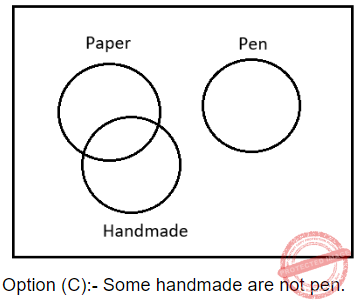Question 147
The proposition 'No historians are non-mathematician' is equivalent to which of the following proposition?
 A All historians are mathematicians B No Historians are mathematicians C Some historians are mathematicians D Some historians are not mathematicians
Logical-Reasoning       Square-of-opposition       UGC-NET June CS 2019 Paper 1
Question 147 Explanation:
(3)=6+5+4+3+2+1=21
(2)=4+3+2+1=10
(3)x(2)=21*10=210
 Question 148
If the proposition 'Houses are not bricks' is taken to be False then which of the following propositions can be TRUE?
(a) All houses are bricks
(b) No house is brick
(c) Some houses are bricks
(d) Some houses are not bricks
Select the correct answer from the options given below:
 A (b) and (c) B (a) and (d) C (b) only D (c) only
Logical-Reasoning       Square-of-opposition       UGC-NET June CS 2019 Paper 1
Question 148 Explanation:
Houses are not bricks ⇒ False
Houses are bricks ⇒ True
→ b and d are not correct.
→ There is no condition that relative to all houses. So, (a) is also incorrect.
→ Only option (c) is correct.
 Question 149
All republics are grateful' and 'Some republics are not grateful' cannot be true, and they cannot both be false. this is called as
 A Contraries B Contradictories C Subaltern D Super altern
Logical-Reasoning       Square-of-opposition       UGC-NET June CS 2019 Paper 1
Question 149 Explanation:
Contradictories opposition is the relation between two propositions having the same subject but differing in both quality and quantity.Relation between A and O and E and I is called contradictory.
A → O
E → I
 Question 150
Identify the reasoning in the following argument: 'Use of teaching aids in the classroom to enhance learning is important in a similar way as that if the use of ICT for production of knowledge'.
 A Hypothetical B Analogical C Inductive D Deductive
Logical-Reasoning       Analogical-Reasoning       UGC-NET June CS 2019 Paper 1
Question 150 Explanation:
A comparison between one thing and another, typically for the purpose of explanation or clarification.
Teaching aids meants for classroom and ICT meant for production of knowledge
 Question 151

Which one of the following hetwabhasa (fallacy) is involved in the argument. “Sound is element because it is caused”?

 A Virudha or contradictory middle B Satpratipakaa or inferentially contradicted middle C Sadhyasama or the unproved middle D Bedhita or non-inferentially contradicted middle
Logical-Reasoning       Fallacies       UGC-NET Dec 2019 Part-1
 Question 152

Consider the following argument:

Statements:
Some chairs are curtains.
All curtains are bedsheets.
Conclusion: Some chairs are bedsheets.

What is the Mood of the above proposition?

 A IAI B IAA C IIA D AII
Logical-Reasoning       Deductive-Inference-and-Syllogism       UGC-NET Dec 2019 Part-1
 Question 153

Which one of the following fallacious hetu (middle term) is not uniformly concomitant with the major term?

 A Asatratipaksa B Auyatireki C Anyonya Asiddha D Suyabicara
Logical-Reasoning       Fallacies       UGC-NET Dec 2019 Part-1
 Question 154

Which of the following is converse of ‘Some S is P’?

 A Some S is not P B Some P is not S C Some P is S D No P is S
Logical-Reasoning       Square-of-opposition       UGC-NET Dec 2019 Part-1
Question 154 Explanation:Question 155

According to classical Indian school of logic, what is the correct sequence of steps involved in Anumana (influence)?

 A Upanaya, Pratijna, Hetu, Udaharaps, Nigmana B Pratijna, Hetu, Upanaya, Udaharana, Nigmana C Pratijna, Upanaya, Hetu, Udaharana, Nigmana D Pratijna, Hetu, Udaharana, Upanaya, Nigmana
Logical-Reasoning       Anumana       UGC-NET Dec 2019 Part-1
There are 155 questions to complete.